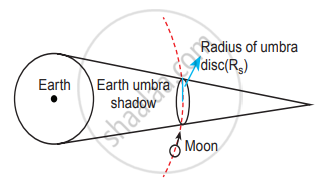Tamil Nadu Board of Secondary EducationHSC Science Class 11th

# Describe the measurement of Earth’s shadow (umbra) radius during total lunar eclipse - Physics

Describe the measurement of Earth’s shadow (umbra) radius during the total lunar eclipse

#### Solution

Lunar eclipse and measurements of the shadow of Earth: On January 31, 2018 there was a total lunar eclipse that was observed from various places including Tamil Nadu. It is possible to measure the radius of the shadow of the Earth at the point where the Moon crosses.Schematic diagram of umbra disk radius

When the Moon is inside the umbra shadow, it appears red in colour. As soon as the Moon Schematic diagram of umbra disk radius exits from the umbra shadow, it appears in a crescent shape.

By finding the apparent radii of the Earth’s umbra shadow and the Moon, the ratio of these radii can be calculated.

The apparent radius of Earth’s umbra shadow = Rs = 13.2 cm

The apparent radius of the Moon = Rm = 5.15 cm

The ratio "R"_"s"/"R"_"m" ≈ 2.56

The radius of Moon Rm = 1737 km

The radius of the Earth’s umbra shadow is Rs = 2.56 × 1737 km ≅ 4446

The correct radius is 4610 km

The percentage of error in the calculation = (4610 - 4446)/4610 xx 100 = 3.5%

The error will reduce if the pictures taken using a high-quality telescope are used.

Concept: Elementary Ideas of Astronomy
Is there an error in this question or solution?

#### APPEARS IN

Tamil Nadu Board Samacheer Kalvi Class 11th Physics Volume 1 and 2 Answers Guide
Chapter 6 Gravitation
Evaluation | Q III. 16. | Page 44
Share